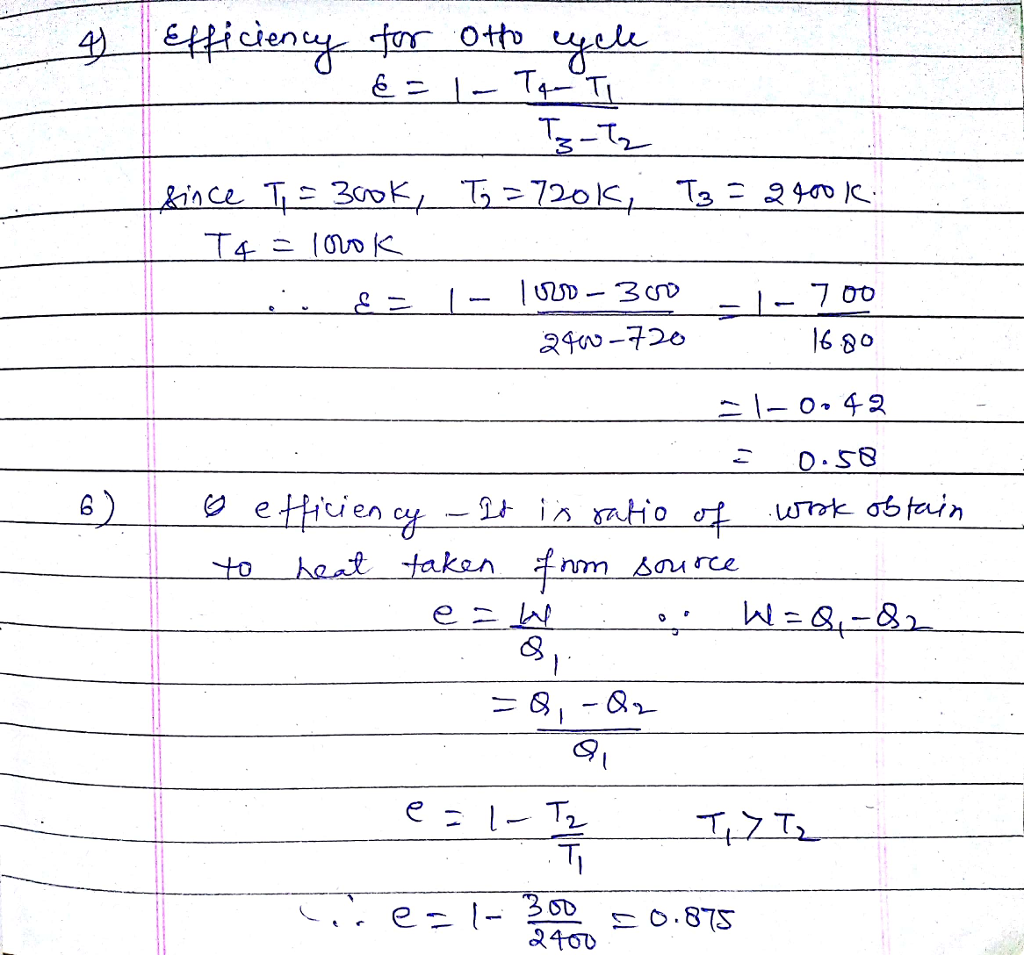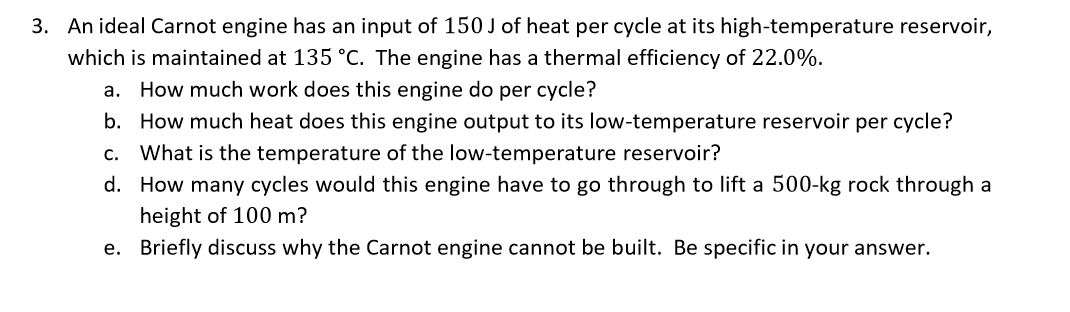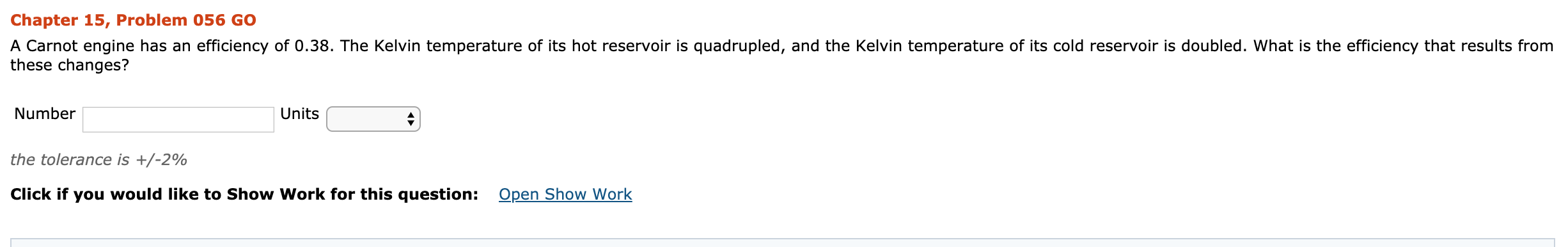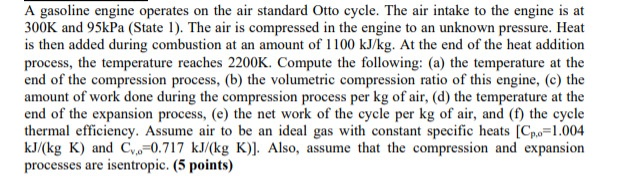Question

# A friend passionate about cars mentions that Toyota has developed an engine with a thermal efficiency...A friend passionate about cars mentions that Toyota has developed an engine with a thermal efficiency of 40% which is an extraordinary accomplishment!

1) Supposing this engine is ideal and based on the Carnot cycle, and also assuming that the cold reservoir temperature is 300 K, what is the hot reservoir temperature to meet the stated efficiency?

You mention your result to this friend who points out correctly that the temperature of the hot reservoir would essentially be the temperature of the burning gasoline/air flame so he doesn't really believe your calculation. You explain the Carnot cycle for an internal combustion engine as seen in class but it doesn't work well because your friend claims that the real thermodynamic cycle in a gasoline engine is the one illustrated below, called the ideal Otto cycle! Note: isentropic means both adiabatic and reversible.

Fearlessly you analyse this thermodynamic cycle to calculate the maximum efficiency and compare with the ideal Carnot cycle

2) Using the diagram on the left, the first law of thermodynamics and the principle that a full cycle of any type of heat engine is associated with zero change in internal energy, show that the thermal efficiency of this ideal Otto cycle is simply given by3) Using the diagram on the right and the relation seen in class between the amount of heat a substance absorbs and how its temperature changes, i.e. Q = Cv*∆T, show that the thermal efficiency of this ideal Otto cycle can also be written as4) Considering T1 = 300 K, T2 = 720 K, T3 = 2400 K and T4 = 1000 K, calculate the thermal efficiency of this ideal Otto cycle.

5) Does it make sense that Toyota was able to build a real world engine (which has various sources of inefficiencies in respect to the ideal Otto cycle) with a thermal efficiency of 40%?

6) If you consider that an ideal Carnot cycle would replace the Otto cycle and that TH = T3 and TC = T1 then what would be the efficiency?#### Earn Coins

Coins can be redeemed for fabulous gifts.

Similar Homework Help Questions
• ### An ideal Carnot thermodynamic engine has an efficiency of 0.600. If it operates between a deep...

An ideal Carnot thermodynamic engine has an efficiency of 0.600. If it operates between a deep lake with a constant temperature of 294 K and a hot reservoir, what is the temperature of the hot reservoir? a. 735 Kelvin b. 490 Kelvin c. 570 Kelvin d. 784 Kelvin

• ### A Carnot engine whose hot-reservoir temperature is 400 ∘C has a thermal efficiency of 45 %...

A Carnot engine whose hot-reservoir temperature is 400 ∘C has a thermal efficiency of 45 % . By how many degrees should the temperature of the cold reservoir be decreased to raise the engine's efficiency to 58 % ?

• ### A Carnot engine has an efficiency of 0.445, and the temperature of its cold reservoir is...

A Carnot engine has an efficiency of 0.445, and the temperature of its cold reservoir is 434 K. (a) Determine the temperature of its hot reservoir. (b) If 5610 J of heat is rejected to the cold reservoir, what amount of heat is put into the engine?

• ### 3. An ideal Carnot engine has an input of 150 J of heat per cycle at...3. An ideal Carnot engine has an input of 150 J of heat per cycle at its high-temperature reservoir, which is maintained at 135 °C. The engine has a thermal efficiency of 22.0%. a. How much work does this engine do per cycle? b. How much heat does this engine output to its low-temperature reservoir per cycle? c. What is the temperature of the low-temperature reservoir? d. How many cycles would this engine have to go through to lift a...

• ### An engine does 20 J of work and exhausts 15 J of waste heat during each cycle. What is the engine's thermal efficiency...

An engine does 20 J of work and exhausts 15 J of waste heat during each cycle. What is the engine's thermal efficiency? If the cold-reservoir temperature is 30 degrees C, what is the minimum possible temperature in degrees C of the hot reservoir?

• ### 3. A newly built engine operates between the room temperature (21 oC) and the melting point...

3. A newly built engine operates between the room temperature (21 oC) and the melting point of silver (961 oC) and claims to have an actual efficiency of 75.0 %. a) How much mechanical energy per cycle does the engine deliver if it absorbs 3.50 x 106 J of heat everycycle (from the hot bath) assuming this claim is true? b) How much thermal energy does the engine reject per cycle to the colder reservoir at 21o C if the...

• ### A Carnot engine operates us ing 1.0 mol e of monoatomic ideal gas as a working...

A Carnot engine operates us ing 1.0 mol e of monoatomic ideal gas as a working s ubstance. In t he first step, the gas is place d in thermal contact with a heat reservoir and expands isothermally to 3 .0 times its initial volume. (a) If the internal energy o f the gas after this step is 6.25 k J , calculate the temperature of the heat reservoir ( T h ) . (b) C alculate the heat absorbed...

• ### help please Chapter 15, Problem 056 GO A Carnot engine has an efficiency of 0.38. The...help please Chapter 15, Problem 056 GO A Carnot engine has an efficiency of 0.38. The Kelvin temperature of its hot reservoir is quadrupled, and the Kelvin temperature of its cold reservoir is doubled. What is the efficiency that results from these changes? Number Units the tolerance is +/-2% Click if you would like to Show Work for this question: Open Show Work

• ### For a Carnot engine with 10 moles of ideal gas (Cv = 1.5 nR) and operating...

For a Carnot engine with 10 moles of ideal gas (Cv = 1.5 nR) and operating between a hot reservoir of 500 K and a cold reservoir of 300 K, a) What would be the heat exchanges (q1) and entropy change (∆S1) for step 1, where the gas reversibly and isothermally expands to double its volume (V2 = 2 V1) at 500 K? b) What would be the heat exchanges (q3) and entropy change (∆S3) for step 3, where the...

• ### A gasoline engine operates on the air standard Otto cycle. The air intake to the engine...A gasoline engine operates on the air standard Otto cycle. The air intake to the engine is at 300K and 95kPa (State 1). The air is compressed in the engine to an unknown pressure. Heat is then added during combustion at an amount of 1100 kJ/kg. At the end of the heat addition process, the temperature reaches 2200K. Compute the following: (a) the temperature at the end of the compression process, (b) the volumetric compression ratio of this engine, (c)...